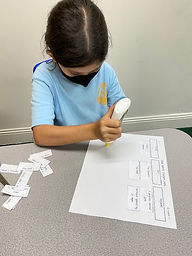Ms. Dawn

Target 1​

Lesson Type:

Continuation

Number Operation

:

Number System

Complete a number sequence from any given integer -100 to 1,000.

1:

Complete a number sequence in both ascending and descending order.

2:

Be able to start a number sequence from any given number.

4th

Vocabulary:

Integers, Sequence, Negative numbers, Positive numbers, Number line, Ascending, Descending, Units

Activities:

1. Students filled in the missing integers on a variety of number lines.

2. Students marked a variety of intergers on a variety of number lines.

3. Students were able to mark integers given a variety of questions (ex. 2 units to the left of 3 is? or 6 units to the right of -1 is?)Home Exploration

Guiding Questions:Absent Students:

Target 2

:

1:

Understand that quantities having a value of more than zero are positive and that quantities having a value of less than zero are negative.

2:

Understand that zero is neither positive nor negative.

3:

Understand that positive and negative numbers occur in the real world.

4:

Understand the relationship between positive and negative numbers.

4th

Vocabulary:

Integer, Real-world, Scenario, Positive, Negative, Zero, Loses, Earned, Owes, Surface, Below, Above, Debit, Sea Level, Neutral, Decrease, Discount

Activities:

1. Students created a list of how positive and negative numbers are used in our real world.

2. Students cut out a variety of real world scenarios and did an integer sort. They needed to place each scenario in the correct place (positive, zero, and negative). Students needed to know the vocabulary to understand where to place each scenario.

3. Students needed to identify integers in real world context. They needed to write an integer to describe each given real-world scenario.Home Exploration

Guiding Questions:Target 3

:

1:

Compare equations which contain both positive and negative numbers.

2:

Compare addition and subtraction equations with both positive and negative numbers.

4th

Vocabulary:

Positive, Negative, Compare, Equations, Integer sign, Operation, Answer sign)

Activities:

1. We discussed the adding positive and negative integers and subtracting positive and negative numbers. We discussed the rules (integer sign, operation, answer sign).

2. Students were given a varitey of positive and negative comparisons. They needed to answer <,>, or =.

3. Students completed a integer operations maze that dealt with addition and subtraction of a varitey of positive and negative numbers.Home Exploration